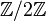# Contractible manifold

This article describes a property of topological spaces obtained as a conjunction of the following two properties: contractible space and manifold

## Definition

A contractible manifold is a topological space satisfying the following equivalent conditions:

1. It is both a contractible space and a manifold.
2. It is both a weakly contractible space and a manifold.

## Examples

Any Euclidean space$\R^n$ for$n$ a nonnegative integer is a contractible manifold. For$n = 1$ or$n = 2$, these are the only contractible manifolds up to homeomorphism. For$n = 3$, however, there exist contractible manifolds (in fact, contractible open subset of$\R^3$), such as the Whitehead manifold, that are contractible but not homeomorphic to Euclidean space.

## Relation with other properties

### Weaker properties

Property Meaning Proof of implication Proof of strictness (reverse implication failure) Intermediate notions
• Any compact connected orientable manifold has top homology equal to$\mathbb{Z}$, making it non-contractible if its dimension is positive.
• If the manifold is not orientable, it has nontrivial fundamental group (in particular, the fundamental group admits$\mathbb{Z}/2\mathbb{Z}$ as a quotient group) and is therefore not contractible.